Document Type : Original Article

Author

M.V. Lomonosov Institute of Fine Chemical Technologies, MIREA - Russian Technological University, Vernadskogo pr., 86, Moscow, 119571, Russia

Abstract

Computer chemistry is a field of science appearing at the intersection of chemistry, mathematics and informatics. For the solution of any task in this field some mathematical representation of chemical structures is need. The most widely used approach to description of molecular structure is based on its representation as a graph G with vertices and edges corresponding to atoms and bonds of molecule. However, there may be other ways of describing the molecular structure. In this paper a number of methods to present the molecular structures of organic compounds (hydrocarbons) as hypergraphs Hk (k=1,2,…) of special type is suggested. Some results of comparison of graph and hypergraph molecular models are also given. Construction of Hk is defined by neighborhoods of k-th order for all vertices in a graph G corresponding to the carbon skeleton of molecule (k=1,2,…). Besides, analytical formulae, expressing the adjacency matrices of Hk throw adjacency matrix of corresponding graph G are obtained (k=1,2,…). The comparison of traditional graph model G and suggested hypergraph models Hk (k=1,2,…) is made by definite quantitative parameters, characterizing the efficiency of their applications in some tasks of computer chemistry. Some 4 different sets of structural formulae of hydrocarbons and 30 different quantitative parameters are used for these investigations. It is shown, that in 97% of all considered 120 cases the model H1 is superior to the model G, and in other cases these models are equivalent. However, the models Hk for k ≥ 2 are worse than G and H1. It is also shown on concrete examples, that in some cases, H1-models may be useful in constructing the structure-property correlations, since their use allows us to obtain more precise correlations than for G-models (in 75% of considered cases).

Graphical Abstract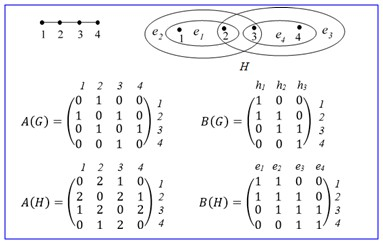Keywords

###### ##### Full Text

Introduction

Computer chemistry is a field of science appearing at the intersection of chemistry, mathematics and informatics. The basic problems of computer chemistry are, in particular, the problems of computer generation of possible reactions between given reagents, the construction of mathematical models connecting the structures and different properties of chemical compounds and prediction the properties of new compounds using this models, computer generation of chemical compounds with prescribed properties, etc. [1-6].

However, for the solution of any task, considered in this field, chemical compounds must be as some mathematical objects represented. Therefore, in computer chemistry, there is a number of special tasks, which deal with constructing the mathematical models of chemical compounds, investigating the properties of these models, elaborating the different algorithms (combinatorial, optimization, etc.) operating with selected mathematical objects.

There are many different types of molecular models; they describe the molecular structure with different level of detailing and reflect certain peculiarities of molecule. However, the most widespread mathematical models of molecules are graphs. These graphs are usually called “molecular graphs”. A molecular graph is a weighted (or labeled) graph with vertices corresponding to atoms of molecule, and with edges corresponding to chemical bonds in it. The weights (or labels) of vertices and edges code atoms and bonds of different chemical nature (see, for example, ).   This graph can be described by matrix A = (aij), where aii is a weight of vertex i, and aij for i ≠ j is a weight of edge (i, j). It is usually supposed that aij = 0 for non-adjacent vertices i and j. For molecules of hydrocarbons, as a rule, simple graphs representing only carbon skeleton of molecule are used. In this case it is assumed that aii = 0, aij = 1 for adjacent vertices i and j, aij = 0 for non-adjacent vertices i and j, and then matrix A is the adjacency matrix of corresponding graph [8, 9].

For each molecular structure, different invariants of its molecular graph may be calculated, that is, the numbers defined by graph, which are independent of numbering the graph vertices. The examples of simple graph invariants are the numbers of vertices, edges, cycles in a graph, determinant of its adjacency matrix, etc. The graph invariants are widely used as so-called molecular descriptors in mathematical models of relation between the structure and properties of chemical compounds; these models are often called Quantitative Structure-Property Relationships or QSPR [8, 10, and 11]. However, graph invariants are not only used in QSPR-analysis, they may be also applied in procedures of coding, ordering, searching for chemical compounds in chemical data bases. Therefore, the problem of search for graph invariants with little degeneration degree (or with big discrimination ability) is of great importance. The investigations of degeneration degrees of graph invariants and comparison of invariants by this parameter on some particular sets of graphs are usually fulfilled (see, for example, ).

For the solution of some problems of computer chemistry the local vertex invariants (LVI) are also used; for example, in the searching the canonical numbering the molecular graph vertices, or in the searching the symmetry group of a graph, or in establishing the graph isomorphism (see, for example, [13, 14]). All methods of solutions of these problems are based on exhaustive search with using some criteria for rejection of unsuitable variants. As a rule, these criteria are formulating in terms of some LVI. For effective solutions of such tasks, it is important to find LVI, which can quantitatively distinguish non-equivalent vertices of a graph; that is, vertices belonging to different orbits of symmetry group of a graph. Therefore, the problem of searching the LVI having the greatest ability to distinguish non-equivalent vertices is actual one. Note that there are other possible applications of LVI, for example, constructing on their base new graph invariants or graph codes, that is, sequences of ordered LVI .

As it is known, a generalization of a notion “graph” is a notion of “hypergraph” [15-20]. A hypergraph H = (V, E) is a pair of some sets V = {v1,…,vn} and E = {e1,…,ep}, where V is a set of vertices, E is a set of hyperedges; any hyperedge ei is some non-empty subset of set V, which can contain any number of elements. Thus, the graph G is a particular case of a hypergraph H, when any hyperedge ei consists of two vertices, and called by edge. In the picture of hypergraph, their hyperedges are indicated with help of closed curve, inside of which place the corresponding vertices; for hyperedge with two vertices these vertices by a segment of strait line are connected. A hypergraph H may be given by its incidence matrix B = (bij) of dimension nxp, where bij = 1, if vertex vi belongs to hyperedge ej, and bij = 0 in otherwise. The example of hypergraph Н with vertices v1,…, v4 and hyperedges e1 = {v1}, e2 = {v2,v3}, e3 = {v1,v2,v4} and its incidence matrix B is given in Figure 1.

For a hypergraph H the adjacency matrix A = (aij) can be also defined: An element aij for  is equal to a number of hyperedges, containing the pair of vertices i and j, and aii = 0. The definitions of invariants or LVI for a hypergraph are similar to definitions of these notations for a graph. Besides, invariants and LVI for hypergraph one can calculate using some their matrices and known algorithms elaborated for ordinary graphs. It should be noted, that, as a rule, hypergraphs with simple hyperedges are considered; that is, each set ei in this construction is taking into account only once.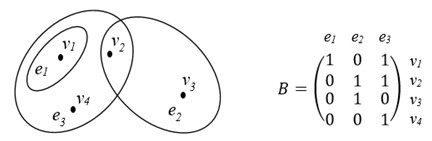Figure 1: Example of hypergraph Н and its incidence matrix В

Hypergraphs are also applying in computer chemistry. Ordinary graphs do not adequately describe chemical compounds of non-classical structures; for example, molecules with delocalized polycentric bonds, in particular, organometallic compounds. In a number of works for description of structures of such compounds the hypergraphs were suggested (see, for example, [19-23]). In a previous study , for very large set of compounds with non-classical structures the graph models and hypergraph models were considered; for these molecular graphs and hypergraphs some known invariants of the same name were calculated and it was shown, that invariants of hypergraphs were essentially less degenerative than analogous invariants of graphs. Some advantages of hypergraph representation of such molecules were also noted. However, in literature any results concerning the using the hypergraph invariants in QSPR-analysis are absent.

To perceive isomorphic hypergraphs, the sanctioned types of rate grids are to be found. The calculation for development of standard rate network of hypergraph is proposed. Some substance issues managing the hypergraph hypothesis are talked about .

Diagram hypothetical ideas are valuable for the portrayal and investigation of communications and connections in organic frameworks .

The consequences of this investigation showed that the affectability of most lists is higher in the hypergraph model. The absolute number of no correlated files additionally increments in the last model .

It should be noted that amount of works devoted to applications of hypergraphs in chemistry is very little, compared with amount of works deals with applications of graphs in chemistry [29, 30]. Besides, the hypergraphs for the description of compounds with classical structures were not used. The main reason of this is that the structural formula of molecule already is a labeled graph, which allows construct on its base other different molecular graphs. Another possible reason of little using of hypergraphs in chemistry is the complexity of visual perception of such graphical constructions.

Material and methods

Note that in all graph models of molecules, two vertices are connected by an edge if and only if there is a chemical bond between the corresponding atoms, indicated in structural formula. However, there is some interaction between atoms, which are not connected by such bonds; in this case, the force of atom interaction depends on distance between atoms. These reasons lead us to idea to assume that “are connected” not two, but a few atoms, which are arranged nearly from each other (by some criteria of nearness) and to use the hypergraphs for description of classical molecular structures. For example, one can assume, that “are connected” all atoms arranged in neighborhood of the 1-st order of fixed atom or all four atoms arranged on a chain of three sequential chemical bonds. Thus, new models of structures of classical compounds arise; these models are hypergraphs and they based on definite interpretation of notion “connectedness of atoms”. The obtained models one can to compare with traditional graph models by some parameters, as this was done, for example, in a series of works [19-23] for the non-classical structures, and to conclude about their appropriateness and usefulness in computer chemistry.

The main goals of the present work were as follows:

1) To elaborate a number of ways of molecular structure description for organic compounds (hydrocarbons) in terms of hypergraphs using for this purpose different interpretations of the notion “connectedness of atoms”;

2) To deduce a general analytical formula, expressing the adjacency matrices of obtained molecular hypergraphs throw the adjacency matrices of corresponding molecular graph;

3) To compare the efficiencies of using the traditional graph model and suggested hypergraph models of molecules in some special tasks of computer chemistry, mentioned above; and,

4) To investigate the usefulness of hypergraph models in constructing the structure-property correlations.

1) A number of ways of constructing the hypergraph models of organic compounds (hydrocarbons of some classes) are suggested.

2) The general analytical formulae connecting the adjacency matrices of obtained hypergraphs and adjacency matrix of initial molecular graph are deduced.

3) A comparison of traditional graph model of hydrocarbons and suggested hypergraph models is by definite quantitative parameters, characterizing the efficiency of their applications in some tasks of computer chemistry. For this comparison, some 4 different sets of hydrocarbons and 30 different quantitative parameters were used. It was found, that one of the suggested hypergraph models in 97% considered cases is superior to the graph model, and in other cases these models are equivalent.

4) It is shown, that in some cases the hypergraph models may be useful in constructing the structure-property correlations, since their use in this process allows us to obtain more precise correlations than for graph models.

Result and Dissection

A set of all 80 structural formulae of saturated hydrocarbons with 6, 7, 8 carbon atoms from , and a set of all 22 structural formulae of alkyl benzenes with 10 carbon atoms from  were used in aforementioned investigations. Molecular graphs of carbon skeletons of all these compounds are given in Figures 2-6. The vertices of pictured graphs correspond to atoms in molecule, and the edges - to chemical bonds between these atoms. We denote these graphs by letter G.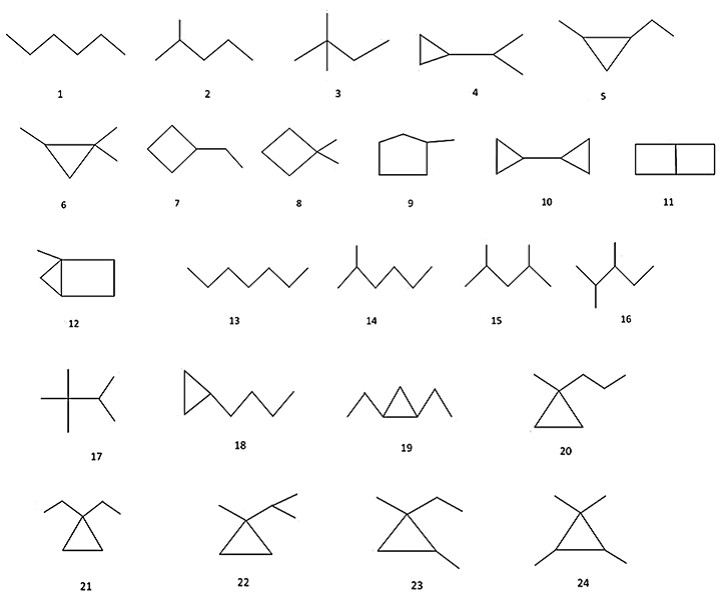Figure 2:  Compounds 1-24 used for construction and investigation of hypergraph molecular models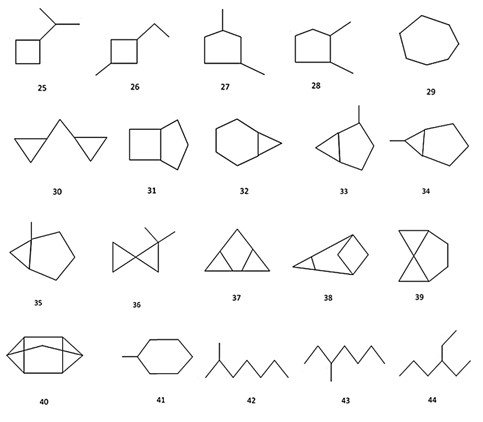Figure 3: Compounds 25-44 used for construction and investigation of hypergraph molecular models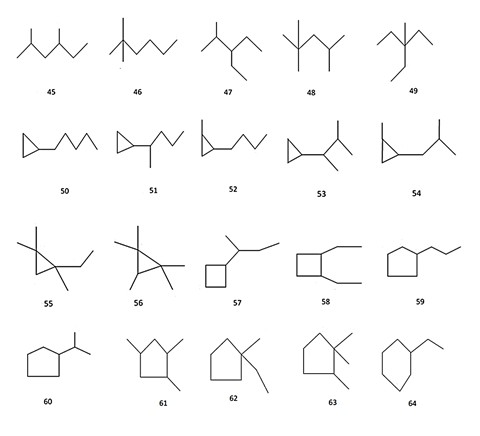Figure 4: Compounds 45-64 used for construction and investigation of hypergraph molecular modelsFigure 5: Compounds 65-80 used for construction and investigation of hypergraph molecular modelsFigure 6: Compounds 81-102 used for construction and investigation of hypergraph molecular models

Let us describe a procedure of constructing Hk-models (k ≥ 1) of aforementioned compounds by initial molecular graphs G, derived from the structural formulae of molecules. Let G = (V (G), E (G)) be a graph with n vertices numbered arbitrarily by numbers 1,…, n. We construct a hypergraph Hk = (V (Hk), E (Hk)), k ≥ 1, by a graph G, using the following rules:

V(Hk )=V(G), E(Hk )={e1,…,ep }, ei={set of vertices j: d(i,j)≤k},                                                                        (1)

Where d (i,j) is the distance between vertices i and j. In other words, hyperedge ei is a neighbor of k-th order of vertex i (i = 1,…, n).

The examples of graph G, hypergraph H = H1, their adjacency matrices A(G), A(H) and their incident matrices B(G), B(H) are given in Figure 7. In this case G = (V (G), E (G)) and H = (V (H), E (H)), where:

V(G) = {1,2,3,4},E(G)={h1, h2, h3},                           (2)

h1 = {1,2}, h2 = {2,3}, h3={3,4};

V(H) = {1,2,3,4}, E(H)={e1, e2, e3 },

e1={1,2}, e2={1,2,3}, e3={2,3,4}, e4={3,4}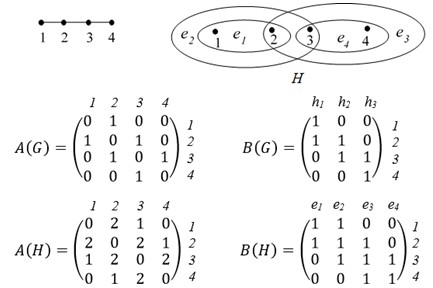Figure 7: Graph G and corresponding hypergraph H = H1 and their matrices A (G), A (H), B (G), B (H)

It should be noted, that if k ≥ D(G), where D(G) is the diameter of a graph G, then all hyperedges of constructed hypergraph Hk will be identical and will coincide with the set V(G). In this case, all elements of matrix B (Hk) will be equal to unit, and all elements of matrix A (Hk) will be equal to n. Thus, all information about a graph structure will be lost and Hk-model will be senseless.

Since in our investigations we compare graph model G and hypergraph models Hk by a number of characteristics of the same name, calculated by their adjacency matrices A (G) and A (Hk), a deduction of analytical relations between A (G) and A (Hk) is interesting.

Let us introduce the following notations: E - identity matrix of dimension n; D (m) = (dij(m)) - symmetrical matrix of dimension nxn, where dij(m) = 1, if dij = m and dij(m) = 0 in otherwise, m = 1,2,…, k; V = (vii) - diagonal matrix of dimension nxn, where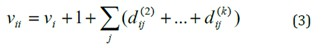vi - degree of vertex I in graph G.

Statement   The following formula, connecting matrices A (G) and A (Hk), k ≥ 1, is true:Proof It is known, that for any hypergraph H (in particular, for any graph G) the following formula takes place: A = BB*-V.  Here A is the adjacency matrix of H (or G), B is the incident matrix of H (or G), B* is the matrix, transposed to B, V = (vii) is the diagonal matrix, vii is the degree of vertex i in H (that is, vii is the number of hyperedges, to which vertex i belongs). In considered case B = B*, hence BB* = B2. Besides, A (G) = D (1) and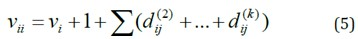Where vi is the degree of vertex i in a graph G. Thus,

 B =E +D(1)+ D(2)+…+ D(k) = E+A(G)+ D2+ D(k) (6)

The required formula follows from this relation. In particular, for k = 1:

A(H) = (E+A(G))2-V = E+2A(G) +A2(G)-V                (7)

Where V is diagonal matrix with diagonal elements, which are equal to vi + 1, where vi is the degree of vertex i in graph G.

Let us define a number of simple local vertex invariants (LVI) of graph and hypergraph, based on their adjacency matrices A.  Let A2 = (aij(2)) be a square of adjacency matrix A of graph G (or hypergraph H). Let us consider the following five LVI:Note, that for graph G the number wi(1) is equal to degree of vertex i, and wi(1) = wi(2) = wi(3). Besides, let us consider 4 following sets of compounds (or corresponding molecular graphs), formed on the base of the set of initial data, consisting of:

• all saturated hydrocarbons with 6 and 7 carbon atoms (№№ 1-41 in Figure 2(a),(b); the number of such compounds is equal to N = 41);
• all saturated hydrocarbons with 8 carbon atoms (№№ 42-80 in Figure 2 (b)-(d); the number of such compounds is equal to N = 39);
• All saturated hydrocarbons with 6, 7, 8 carbon atoms (№№ 1-80 in Figure 2(a)-(d); the number of such compounds is equal to N = 80).
• All alkyl benzenes with 10 carbon atoms (№№ 81-102 in Figure 2(e); the number of such compounds is equal to N =22).

Let us compare LVI of the same name by their capacities to distinguish non-equivalent vertices in initial molecular graphs. For this purpose, two quantitative characteristics of this capacity, N1 and N2, are introduced. The number N1 is a fraction of structures in considered set, in which there are indistinguishable non-equivalent vertices. It is easily to see, that 0 ≤ N1 ≤ 1, and N1 = 0 for the best case, when all non-equivalent vertices in all considered structures are distinguishable, and N1 = 1 for the worst case, when in all structures all non-equivalent vertices are indistinguishable.

Let us now define the second characteristics, N2. At first, we find in each graph G all non-equivalent vertices, which are indistinguishable by considered LVI; these vertices will belong to different classes of equivalence and will have the same values of LVI. Then, we find the minimal number of vertices in this set satisfying the following condition: If we change, by some way, the values of their LVI, then all non-equivalent vertices in a given graph will be distinguishable. Let N2 is a total number of such vertices in all graphs of considered set, M is total number of vertices in these graphs, N is total number of graphs in considered set. It is easily to see, that 0 ≤ N2M-N. Define N2 by the following way:Evidently, 0 ≤ N2 ≤ 1, and for the best case N2 = 0, and for the worst case N2 = 1, when all vertices in a graph have the same values of LVI, and it is takes place for any graph in considered set). It is natural to accept that the best model from several ones is that which has the least value of N1 (or N2).

In Tables 1 and 2, the obtained values of N1 and N2 are given for all 5 variants of LVI for 4 sets of compounds (1)-(4), for G and H = H1. From these tables, it follows that in 38 considered cases (from 40 ones) H1-model is better than G-model; that is, the values of N1 and N2 for H1 are less than corresponding values for G; and in 2 cases these models are equivalent. However, for considered sets of compounds Hk-models for k > 1 are worse than H1-model and G-model.Let us define codes Kj(G) and Kj(H) (j = 1,…,5) for graphs and hypergraphs as the sequences of LVI wi(j) (j = 1,…,5) arranged in ascending order. For example, K1(G) = {1,1,1,2,3,4}, K1(H) = {4,5,5,9,10,11} for graph G and hypergraph H, as shown in Figure 3.

Let us compare these codes by their degeneration degrees. For this purpose, we introduce the quantitative characteristics of degeneration degree of a code, N3 = N3’/N, where N3is the number of different codes for given set of structures. Evidently, 1/N ≤ N3 ≤ 1, and in the best case N3 = 1, in the worst case N3 = 1/N. In Table 3 obtained values of N3 for the sets of molecular graphs (1)-(4) for H1- and G-models are given.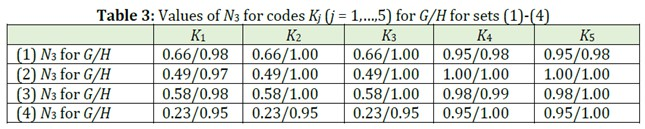It follows from these data that in 18 cases (from 20 ones) H1-model is better than G-model (that is, N3 for H1-model is more than N3 for G-model); in 2 cases both models are equivalent (values of N3 for both models are the same). Besides, H1-model in 11 cases gives “ideal” result (N3 = 1). However, for considered structures Hk-models for k > 1 are worse than H1 -and G-models.

Let us consider the following 15 simple invariants for graphs and hypergraphs, constructed on the bases of LVI introduced above and on the base of spectral characteristics of adjacency matrix A:

I1 = min wi(1) I2 = max wi(1), I3 = min wi(3), I4 = max wi(3),

I5 = min wi(4),  I6 = max wi(4), I7 = min wi(5), I8 = max wi(5),

I9 = S1 = Σ wi(1)I10  = S2 = Σ (wi(1))2,

I11 = λmax ─ maximal eigenvalue of matrix A,

I12 = λmin  ─ minimal eigenvalue of matrix A,

I13 =  λmax - λminI14 = detA ─ determinant of matrix A,  I15 =  λmax + λmin

Let us compare the degeneration degrees of these invariants on the sets (1)-(4). For this purpose, we will use quantitative characteristic N3 (see subsection 3.2). In Tables 4-6 the obtained values of N3 for the sets (1)-(4) and all 15 described above invariants for G and H = H1 are given.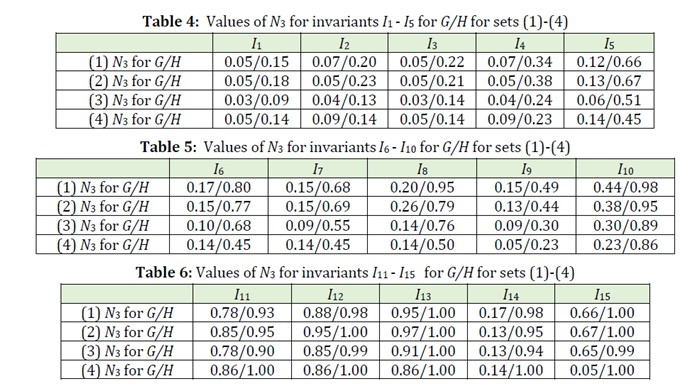It follows from these data that in all 60 cases H1-model is better than G-model (that is, N3 for H1-model is more than N3 for G-model). Besides, H1-model in 11 cases gives “ideal” result (N3 = 1).  However, for considered structures Hk-models for k > 1 are worse than H1- and G-models.

In this section we consider the set of 22 alkylbenzenes (structures  Compounds 81-102, Figure 2(e)) with known values of a number of physico-chemical properties (boiling point, bp (°C); density, d (g×cm-3); refractive index, nD20; heat of combustion, DHc (kcal×mol-1)), given in Table 7 . Using these data, we will analyze the usefulness of H-models in constructing the structure-property correlations.

The values of invariants I1-I15 (see subsection 3.3) for these structures were earlier calculated for G -models and H-models. Let us denote by symbols {I(G)}and {I(H)}the sets of obtained invariants, that is {I(G)}={I1(G),…,I15(G)} and  {I(H)}={I1(H),…,I15(H)}. We construct for each property under consideration the structure-property correlations with the best 2, 3, 4 parameters, choosing them independently from the one with the following 3 sets:

a){I(G)};        b) {I(H)};      c) {I(G)}U{I(H)}.

For each correlation, we found mean square deviation s and correlation coefficient R; we will further use these parameters to estimate the quality of correlations and to compare one correlation with another. In Tables 8-11 the best 2, 3, 4 parameters for all these cases and corresponding s and R are given.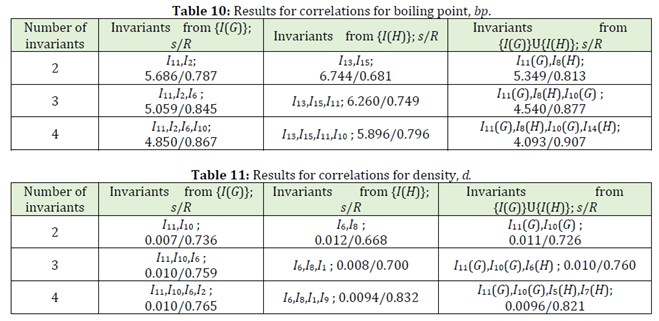Let us analyze the obtained results for all 12 correlations, comparing s and R for correlations for the sets a), b), c) in analogous cases. As we can see, in 9 cases from 12 ones (that is, in 75% of all considered cases) the use of invariants of hypergraphs allows us to obtained more precise correlations than correlations with invariants from the set {I(G)} only.

Thus, in a number of cases the hypergraph models may be useful in constructing the structure-property correlations.

Conclusion

In the present paper, a number of ways of constructing the hypergraph models Нk (k ≥ 1) of organic compounds (hydrocarbons of some classes) are described. The vertices of Нk correspond to carbon atoms in molecule, and each hyper edge in Нk is defined as a set of vertices belonging to the neighborhood of k-th order of some fixed vertex for constructing the hyper edges all vertices are considered.

Besides, the analytical formulae connecting the adjacency matrices of obtained hypergraphs Нk (k ≥ 1) and adjacency matrix of initial molecular graph G are deduced; these dependences are nonlinear ones.

A comparison of traditional graph model G and suggested hypergraph models Нk (k ≥ 1) is made by definite quantitative parameters, characterizing the efficiency of their applications in some tasks of computer chemistry. For this comparison, some 4 different sets of hydrocarbons, presented by their structural formulae, and 30 different quantitative parameters are used. It is shown that in 116 cases from considered 120 ones the model H1 is superior to the model G (that is, in 97% of all cases), and in other cases these models are equivalent.  However, for considered sets of structures, the models Hk for k > 1 are worse than the models H1 and G; the reason for this, probably, is relatively small sizes of considered molecules.  Analyzing the results obtained, we can conclude that the hypergraph molecular models can be successfully used in computer chemistry instead traditional graph models.

It is also shown on concrete examples, that in some cases, the hypergraph models may be useful in constructing the structure-property correlations; since their use in this process allows us to obtain more precise correlations than for G-models; this takes place in 75% of all considered cases.

Funding

This research did not receive any specific grant from funding agencies in the public, commercial, or not-for-profit sectors.

Authors' contributions

All authors contributed toward data analysis, drafting and revising the paper and agreed to be responsible for all the aspects of this work.

Conflict of Interest

We have no conflicts of interest to disclose.

Maria Skvortsova. Molecular Graphs and Molecular Hypergraphs of Organic Compounds: Comparative Analysis, J. Med. Chem. Sci., 2021, 4(5) 452-465

DOI: 10.26655/JMCHEMSCI.2021.5.6

###### ##### References
1. Marsili M., Computer Chemistry. CRC Press, Boca Raton. 1990 [Crossref], [Google scholar], [Publisher]
2. Solov’ev M.E., Solov’ev M.M., Computer Chemistry. SOLON-Press, Moscow. 2005 [Google scholar]
3. Basak S.C., Restrepo J., Villaveces J.L., Advances in Mathematical Chemistry and Applications. Elsevier-Bentham, Sharjah. 2014 [Crossref], [Google scholar], [Publisher]
4. Wagner S., Wang H., Introduction to Chemical Graph Theory. Chapman and Hall/CRC, London. 2018 [Crossref], [Google scholar], [Publisher]
5. Bonchev D., Rouvray D.H., Chemical Graph Theory: Introduction and Fundamentals. Routledge, London. 1991 [Google scholar], [Publisher]
6. Kerber A., Laue R., Meringer M., Rücker C., Schymanski E., Mathematical Chemistry and Chemoinformatics. De Gruyter, Berlin. 2014 [Crossref], [Google scholar], [Publisher]
7. Schulaeva N.A., Skvortsova M.I., Mikhailova N.A., Fine Chem. Technol., 2020, 15:84 [Crossref], [Google scholar], [Publisher]
8. Stankevitch M.I., Stankevitch I.V., Zefirov N.S., Chem. Rev., 1988, 57:191 [Google scholar], [Publisher]
9. Sabzi A.H., Dana A., Salehian M.H., Shaygan Yekta H., J. Pediatr., 2021, 9:13671 [CrossRef], [Google Scholar], [Publisher]
10. Todeschini R., Consonni V., Handbook of Molecular Descriptors. Wiley-VCH, Weinheim. 2000 [Crossref], [Google scholar], [Publisher]
11. Devillers J., Balaban A.T., Topological Indices and Related Descriptors in QSAR and QSPR. Gordon and Breach, Amsterdam. 1999 [Crossref], [Google scholar], [Publisher]
12. Rücker G., Rücker C., Chem. Inf. Comput. Sci., 1999, 39:788 [Crossref], [Google scholar], [Publisher]
13. Stankevich I.V., Gal’pern E.G., Chistyakov A.L., Baskin I.I., Skvortsova M.I., Zefirov N.S., Tomilin O.B., Chem. Inf. Comput. Sci., 1994, 34:1105 [Crossref], [Google scholar], [Publisher]
14. Stankevitch M.I., Tratch S.S., Zefirov N.S., Comput. Chem., 1988, 9:303 [Crossref], [Google scholar], [Publisher]
15. Zykov A.A., Math. Surv., 1974, 29:89 [Google scholar], [Publisher]
16. Bretto A., Hypergraph Theory. Springer, New York. 2013 [Google scholar], [Publisher]
17. Voloshin V.V., Introduction to Graph and Hypergraph Theory. Nova Science Publishers, New York. 2009 [Google scholar], [Publisher]
18. Seyyedrezaei S.H., Khajeaflaton S., Ghorbani S., Dana A., J. Pediatr., 2021, 9:12775 [CrossRef], [Google Scholar], [Publisher]
19. Konstantinova E.V., Skorobogatov V.A., Struct. Chem., 1998, 39:268 [Crossref], [Google scholar], [Publisher]
20. Konstantinova E.V., Skorobogatov V.A., Struct. Chem., 1998, 39:958 [Crossref], [Google scholar], [Publisher]
21. Konstantinova E.V., Skorobogatov V.A., Chem. Inf. Comput. Sci., 1995, 35:472 [Crossref], [Google scholar], [Publisher]
22. Konstantinova E.V., Chemical hypergraph theory. Pohang Univ. Sci. Tech., Pohang. 2001 [Google scholar]
23. Konstantinova E.V., Skorobogatov V.A., Math.,2001, 235:365 [Crossref], [Google scholar], [Publisher]
24. Skvortsova M.I., Fedyaev K.S., Palyulin V.A., Zefirov N.S., Internet Electron. J. Mol. Des., 2003, 2:70 [PDF], [Google scholar], [Publisher]
25. Obolentsev R.D., Physical Constants of Hydrocarbons of Liquid Fuels and Oils. Gostoptekhizdat, Moscow-Leningrad. 1953 [Google scholar]
26. Konstantinova, E.V., Skorobogatov V.A., Math.,2001, 235:365 [Crossref], [Google scholar], [Publisher]
27. Huber W., Carey V.J., Long L., Falcon S., Gentleman R., BMS Bioinform., 2007, 8:1 [Crossref], [Google scholar], [Publisher]
28. Konstantinova E.V., Skoroboratov V.A., Struct. Chem., 1998, 39:958 [Crossref], [Google scholar], [Publisher]
29. Gözükızıl M. F., Chem. Bull., 2020, 9:335 [Google scholar]
30. Chachkov D.V., Mikhailov O.V., Chem. Bull., 2020, 9:9 [Google scholar]